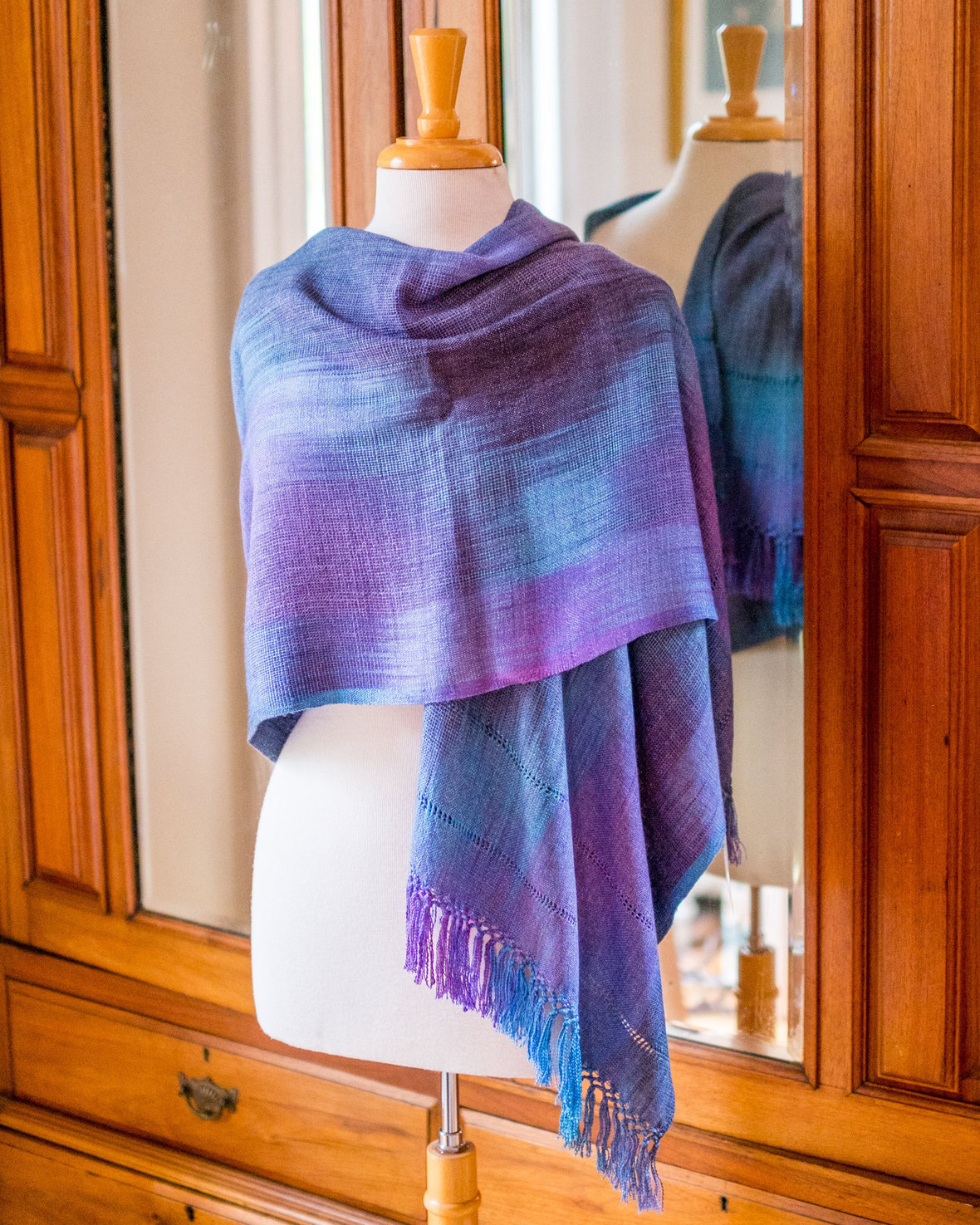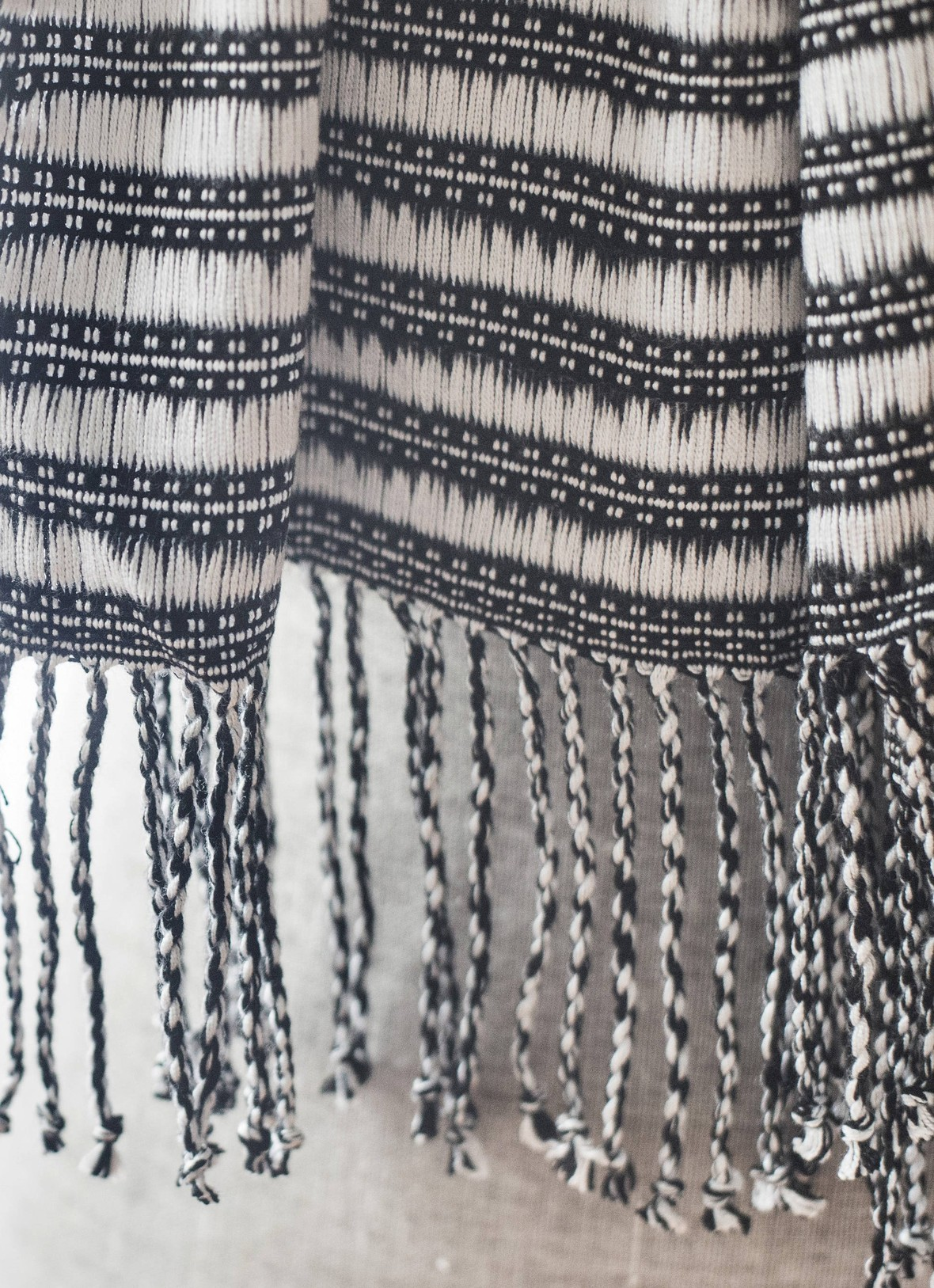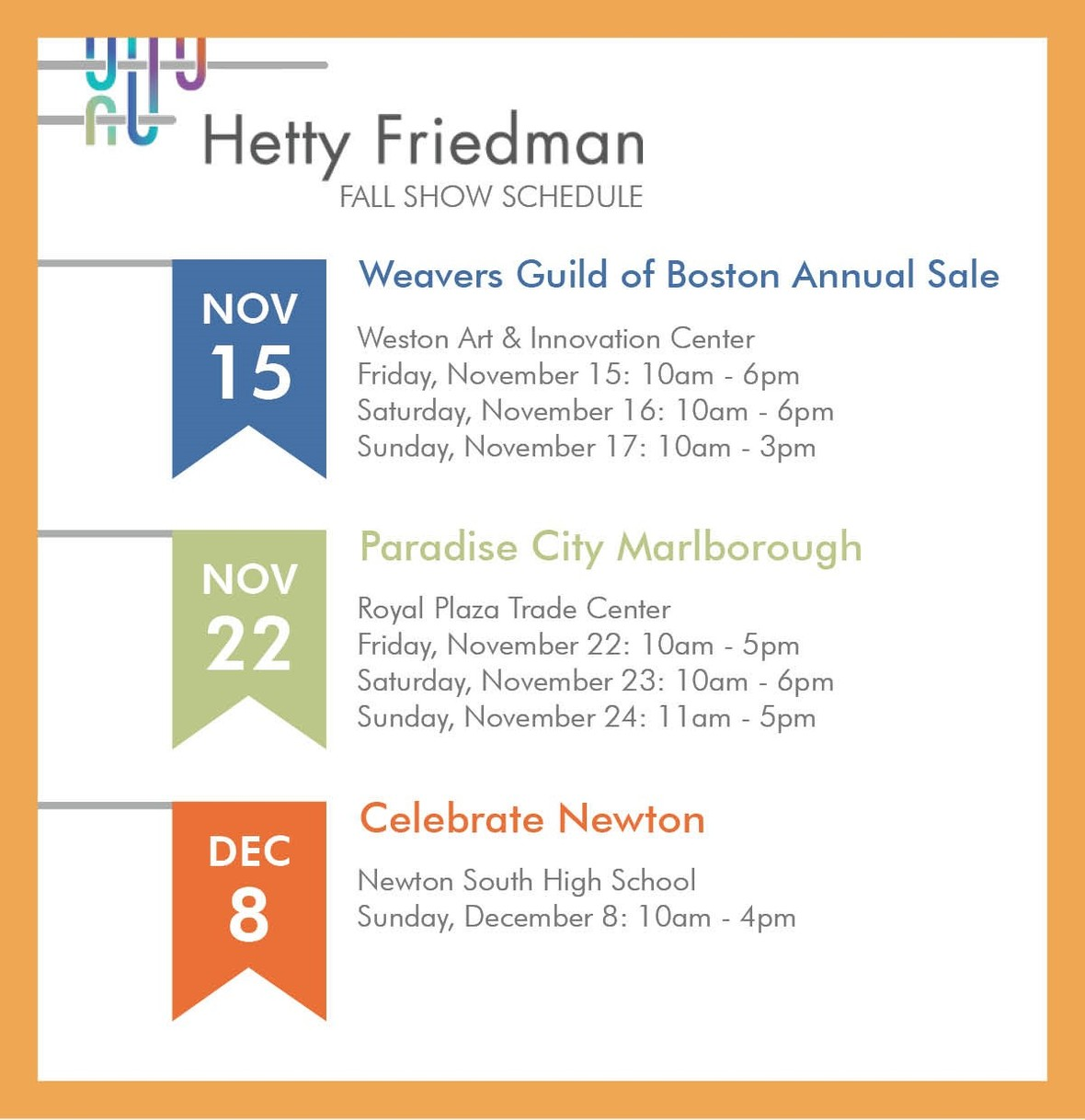Like   +1   in# I'm sharing my summer book list.

These are some of my recent reads. Maybe some of them will suit your fall reading needs. What is on your bedside stand? Share them with us.

 table div table+table+table div table{width:100%;padding:0}table div table+table+table div table table{padding:0;float:left!important;width:53.962%!important}table div table+table+table div table table+table td,table div table+table+table div table td{padding-left:0;padding-right:0}table div table+table+table div table table td{padding-left:0;padding-right:20px}table div table+table+table div table table+table{float:left!important;width:46.038%!important}/* styles */
 /* styles */ A Few Feet Short by Jamey Glasnovic This one surprised me. I grabbed it from the librarian recommendations. It's a quirky travel log. I could relate to Jamey's trials as he tramps around Nepal. The Genius of Birds by Jennifer Ackerman About 10 years ago I did my first bird watching walk in Guatemala. Since then, I've discovered some of the incredible pleasures in making distinctions about what I see. I'm not a big non-fiction reader but this was recommended as a book club selection from NPRs Science Friday. I loved it. Give it a try. Home Fire by Kamila Shamsie Came to me from my daughter, Michaela. 'Home Fire' is set among two Pakistani émigré families living in very different communities in London. It's interesting, passionate and hard to put down.
 table div table+table+table+table+table div table,table.module-4{width:50.19%;float:left;padding:0}table div table+table+table+table+table div table a{border:0 none;text-decoration:none}table div table+table+table+table+table div table img{width:100%!important;border:0 none;text-decoration:none}table div table+table+table+table+table div table td{width:100%;padding:0 20px 20px 0}/* styles */ Becoming Michelle Obama by Michelle Obama This book exceeded my expectations. If you haven't read it yet, do so. Lord of Scoundrels by Loretta Chase My good friend Loretta Chase (aka Clark U friend), really writes a great book. Perfect for holing up under the covers or a long plane ride. The Underground Railroad bt Colson Whitehead Another from Michaela's list. It wasn't an easy read but I learned a lot. Take it in pieces. It's a must read.
 /* styles */ When Breath Becomes Air by Paul Kalanithi This is a non-fiction memoir published posthumously. Some great philosophy. Half of a Yellow Sun by Chimamanda Ngozi AdichieI I lived through the Biafran War but to be honest I didn't take it in. This novel is captivating and will open your mind to what happened. Wonderfully written.
 table div table+table+table+table+table+table+table div table{width:100%;padding:0}table div table+table+table+table+table+table+table div table table{padding:0;float:left!important;width:55.471%!important}table div table+table+table+table+table+table+table div table table+table td,table div table+table+table+table+table+table+table div table td{padding-left:0;padding-right:0}table div table+table+table+table+table+table+table div table table td{padding-left:0;padding-right:20px}table div table+table+table+table+table+table+table div table table+table{float:left!important;width:44.529%!important}/* styles */
 table div table+table+table+table+table+table+table+table div table{width:100%;padding:0}table div table+table+table+table+table+table+table+table div table img{width:96.23%;padding:0;float:none}table div table+table+table+table+table+table+table+table div table td{width:100%;padding:0 1.88% 18px}/* styles */# 10% off all rectangle shawls and rayon scarves!

## Offer ends October 31st. Treat yourself to a brand new fall accesory.This elegant hand dyed organic bamboo shawl is in dynamic tones of blue, purple, denim, lavender.This soft, drapey scarf is a great basic to match everthing in your wardrobe.

## You have two weeks--don't miss out!

 table div table+table+table+table+table+table+table+table+table+table+table+table+table div table{width:100%;padding:0}table div table+table+table+table+table+table+table+table+table+table+table+table+table div table img{width:96.23%;padding:0;float:none}table div table+table+table+table+table+table+table+table+table+table+table+table+table div table td{width:100%;padding:0 1.88% 18px}/* styles */# Come say hello!table div table+table+table+table+table+table+table+table+table+table+table+table+table+table+table+table div table{width:100%;padding:0}table div table+table+table+table+table+table+table+table+table+table+table+table+table+table+table+table div table img{width:96.23%;padding:0;float:none}table div table+table+table+table+table+table+table+table+table+table+table+table+table+table+table+table div table td{width:100%;padding:0 1.88% 18px}/* styles */# Don't miss a thing.

Click the buttons below to get all the latest behind-the-scenes photos, thought provoking articles, and special offers. And thank you for sharing my newsletter.

 table div table+table+table+table+table+table+table+table+table+table+table+table+table+table+table+table+table+table div table{width:100%;padding:0}table div table+table+table+table+table+table+table+table+table+table+table+table+table+table+table+table+table+table div table table{padding:0;float:left!important;width:34.716%!important}table div table+table+table+table+table+table+table+table+table+table+table+table+table+table+table+table+table+table div table table+table+table td,table div table+table+table+table+table+table+table+table+table+table+table+table+table+table+table+table+table+table div table td{padding-left:0;padding-right:0}table div table+table+table+table+table+table+table+table+table+table+table+table+table+table+table+table+table+table div table table td,table div table+table+table+table+table+table+table+table+table+table+table+table+table+table+table+table+table+table div table table+table td{padding-left:0;padding-right:20px}table div table+table+table+table+table+table+table+table+table+table+table+table+table+table+table+table+table+table div table table+table{float:left!important;width:34.528%!important}table div table+table+table+table+table+table+table+table+table+table+table+table+table+table+table+table+table+table div table table+table+table{float:left!important;width:30.756%!important}/* styles */
 table div table+table+table+table+table+table+table+table+table+table+table+table+table+table+table+table+table+table+table div table{width:100%;padding:0}table div table+table+table+table+table+table+table+table+table+table+table+table+table+table+table+table+table+table+table div table img{width:96.23%;padding:0;float:none}table div table+table+table+table+table+table+table+table+table+table+table+table+table+table+table+table+table+table+table div table td{width:100%;padding:0 1.88% 18px}/* styles */# Have you forgotten all the ways to wear your mobius shawl?

## Check out this video and pass it along to your friends!

 table div table+table+table+table+table+table+table+table+table+table+table+table+table+table+table+table+table+table+table+table+table div table{width:100%;padding:0}table div table+table+table+table+table+table+table+table+table+table+table+table+table+table+table+table+table+table+table+table+table div table img{width:96.23%;padding:0;float:none}table div table+table+table+table+table+table+table+table+table+table+table+table+table+table+table+table+table+table+table+table+table div table td{width:100%;padding:0 1.88% 18px}/* styles */## Visit my website: hettyfriedmandesigns.com

Email: hetty.friedman@gmail.com Phone: 617.512.5344